# AFPT – Basic Usage

#### 2020-03-18

library('afpt')
1. Define a bird description using the Bird() constructor.
2. Compute flight performance using computeFlightPerformance().

## Bird description

A bird can be defined using the Bird() construction function. This function ensures that the generated object contains all required parameters that describe the bird.

myBird <- Bird(
massTotal = 0.215, #  weight in kg
wingSpan = 0.67,  #  wingspan in m
wingArea = 0.0652, #  wing area in m2
name = 'Jackdaw',
name.scientific = 'Corvus monedula',
type = 'passerine',
source = 'KleinHeerenbrink M, Warfvinge K and Hedenstrom A (2016) J.Exp.Biol. 219: 10, 1572--1581'
)

Any parameters that are not specified, but that are required by any of the flight performance calculations will be filled in with either defaults or estimated with allometric scaling relationships. For instance, the body frontal area, that is used for computing body drag, is in this case calculated based on the specified massTotal from an allometric relationship for type='passerine':

myBird$bodyFrontalArea ##  0.005020037 It is possible to modify the bird description simply by reassigning the variables in the list, i.e. myBird$wingbeatFrequency <- 5

However, it should be noted that some of the variables are related to others (e.g. massTotal is the sum of massEmpty, massFat and massLoad), so that manual changes can lead to unexpected results.

The default bird settings mostly follow the suggestions in Pennycuick (2008).

## Compute flight performance

Flight performance can be computed using computeFlightPerformance(). It calculates maximum available mechanical power from the muscles, aerodynamic (mechanical) power for flapping flight as a function of airspeed and the corresponding chemical power.

flightperf <- computeFlightPerformance(myBird)
flightperf
## Name: Jackdaw
## Sc. name: Corvus monedula
## Bird definitions: KleinHeerenbrink M, Warfvinge K and Hedenstrom A (2016) J.Exp.Biol. 219: 10, 1572--1581
##               speed power.aero power.chem strokeplane amplitude
## minimumSpeed  3.398      4.532      23.93        49.9      53.6
## minimumPower  8.144      2.167      12.62        25.3      37.6
## maximumRange 11.110      2.603      14.71        16.9      40.2
## maximumSpeed 15.433      4.531      23.93        10.6      51.2
## Maximum climb performance:
##                  speed power.aero power.chem strokeplane amplitude climbRate
## maximumClimbRate 8.886      4.531      23.93        22.6      53.5    0.8587
## Minimized migration time:
##                  speed speed.migration power.aero power.chem power.dep
## minimumTimeSpeed 11.82           1.934      2.819      15.74     3.081
##                  strokeplane amplitude
## minimumTimeSpeed       15.55     41.62

This is the simplest call to computeFlightPerformance(). The print() of the flightperf shows a summary of the computed powercurve. The function computeFlightPerformance() has calculated several characteristic airspeeds:

• minimumSpeed: the required aerodynamic power equals the maximum available muscle power1.
• minimumPower: the required aerodynamic/chemical power is minimal, i.e. this is the speed for maximum endurance.
• maximumRange: the cost of transport is minimal, i.e this is the speed for maximum range.
• maximumSpeed: required aerodynamic power equals the maximum available muscle power.

These characteristic speeds are stored as a data.frame named table, which contains several more advanced variables not shown in the print, as explained in the following sections.

The function computeFlightPerformance() also estimates the best climb performance, i.e. the maximum climb rate that can be achieved given the power available from the muscles. This data point is stored in the data.frame named maxClimb. It contains the same variables as table, plus an additional one: climbRate. Similarly, the function also produces a data.frame named minTime, which holds the flight performance for time minimizing migratory flight. At this airspeed, which will be slightly higher than the maximum range speed, the bird balances flight time with the time it takes to (re)gain the energy lost during flight.

Finally, computeFlightPerformance() also computes a powercurve for a range of speeds. The length of this curve can be specified by providing a length.out argument, but the default produces 10 points. This curve can be used to visualize the speed dependency of the various variables.

powercurve <- flightperf$powercurve plot(flightperf,symbol.speed="U") plot(powercurve$speed,powercurve$amplitude,xlab=NA,ylab=NA,type='b',xlim=c(0,20),ylim=c(20,60)) mtext(side = 1, line = 2, 'Airspeed (m/s)') mtext(side = 2, line = 2, 'Peak amplitude (deg)')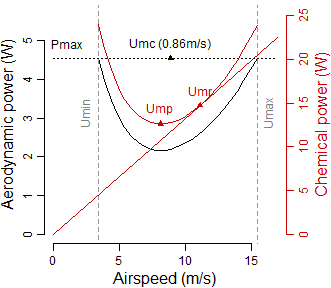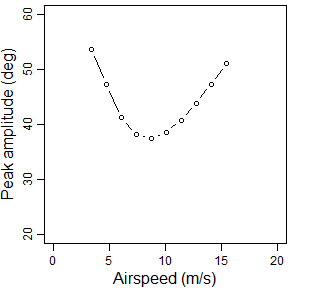### Wingbeat kinematics In the current example, the strokeplane is optimized. The model also implicitly optimizes the wingbeat amplitude for minimum induced power, so essentially the returned powercurve represents the optimal wingbeat for a given wingbeat frequency. flightperf$table[c('speed','frequency','strokeplane','amplitude')]
##                  speed frequency strokeplane amplitude
## minimumSpeed  3.397602         5    49.94069  53.63391
## minimumPower  8.144311         5    25.30698  37.55072
## maximumRange 11.109520         5    16.89529  40.18648
## maximumSpeed 15.433460         5    10.60542  51.16479

By default, computeFlightPerformance() will try to optimize the strokeplane angle for minimum power at any given speed. This optimization is performed many times, which results in a high computational load. The optimization can be avoided by explicitly specifying a strokeplane angle, e.g. computeFlightPerformance(myBird,strokeplane=0).

### Validity flags

table contains flags that indicate whether the data point is outside the validity range of the model:

flightperf$table[,grep('^flags.',names(flightperf$table))]
##              flags.redFreqLo flags.redFreqHi flags.thrustHi flags.speedLo
## minimumSpeed           FALSE            TRUE           TRUE         FALSE
## minimumPower           FALSE           FALSE          FALSE         FALSE
## maximumRange           FALSE           FALSE          FALSE         FALSE
## maximumSpeed           FALSE           FALSE          FALSE         FALSE

In this case the flags indicate the the minimum speed is outside the model’s validity range, indicating too high reduced frequency and too high thrust requirement. The model was obtained for reduced frequencies $$1 \leq k_f \leq 6$$ and thrust requirements (thrust to lift ratio) $$0 \leq \frac{T}{L} \leq 0.3$$. The flag flags.speedLo indicates that the data point is below the low speed limit of the model ($$U<2w_\mathrm{i,h}$$; airspeed is lower than twice the induced downwash in hover2). Data points with flags may be reasonable extrapolations of the model, but it is safer to assume flagged data points are invalid.

### Non-flapping drag components

Stored in the table are also the drag components. Only the non-flapping (Dnf) components are stored:

flightperf$table[,grep('^Dnf.',names(flightperf$table))]
##                 Dnf.ind    Dnf.pro0   Dnf.pro2     Dnf.par
## minimumSpeed 0.44582824 0.008148623 0.28929485 0.007098852
## minimumPower 0.07758968 0.030241749 0.05034741 0.040789816
## maximumRange 0.04169866 0.048180164 0.02705797 0.075898565
## maximumSpeed 0.02160658 0.078889703 0.01402036 0.146477065

These are the induced drag, zero lift profile drag, lift dependent profile drag and parasitic drag, respectively. The latter includes the body drag, but also any other additional drag (e.g. the drag component of weight during climb).

par(mar=c(3.1,3.1,0.4,1.1),mgp=c(1.9,.7,0),cex=0.75)
with(powercurve , plot( speed, Dnf.ind, type='b', col='red3',
xlab=NA, ylab=NA, xlim=c(0,20), ylim=c(0.0,0.20)))
with(powercurve , lines( speed, Dnf.pro0, type='b', col='green3'))
with(powercurve , lines( speed, Dnf.pro2, type='b', col='blue3'))
with(powercurve , lines( speed, Dnf.par, type='b', col='yellow3'))
mtext(side = 1, line = 2,'Airspeed (m/s)')
mtext(side = 2, line = 2,'Drag (N)')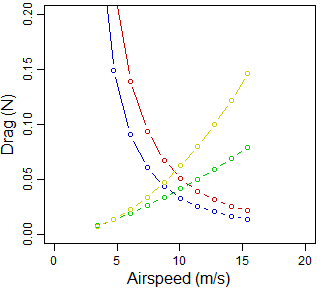Non-flapping drag components – Red: induced drag; green: zero-lift profile drag; blue: lift-dep. profile drag; yellow: parasitic drag;

The above figure shows the magnitude of the non-flapping drag components for different speeds. The lift dependent components are very large at low speeds and they decrease rapidly with increasing speed. The parasitic drag shows the classic quadratic behaviour with speed. The zero-lift profile drag is near zero at low speeds, and increases more linearly with speed. This is due to the strong dependence of the friction coefficient on the Reynolds number. As non-flapping effectively means gliding, these drag components can be used to construct a drag polar for gliding flight. However, this ignores the wing flex that typically occurs at higher speeds.

### Drag factors and power factors

Flapping the wings alters the drag experienced by the wings: $$D = k_D D^\prime$$ (using $$D^\prime$$ for non-flapping drag).3 The factors $$k_D$$ depend on the wingbeat kinematics and are returned in table:

flightperf$table[,grep('^kD.',names(flightperf$table))]
##                kD.ind   kD.pro0   kD.pro2
## minimumSpeed 0.942585 2.4759503 0.7855803
## minimumPower 1.327851 1.0184287 1.2634913
## maximumRange 1.466031 0.9736113 1.4052916
## maximumSpeed 1.875661 0.9167451 1.7971466

These factors are for induced drag, zero lift profile drag and lift dependent profile drag respectively.

The aerodynamic power components for flapping flight can be computed in a similar way: $$P = k_P D^\prime U$$. The factors $$k_P$$ depend on the wingbeat kinematics and are also stored in table:

flightperf$table[,grep('^kP.',names(flightperf$table))]
##                kP.ind   kP.pro0  kP.pro2
## minimumSpeed 1.928459 2.8711163 1.533473
## minimumPower 1.554799 1.0547097 1.444999
## maximumRange 1.636867 0.9964092 1.557558
## maximumSpeed 2.045005 0.9506620 1.990849
par(mar=c(3.1,3.1,0.4,1.1),mgp=c(1.9,.7,0),cex=0.75)
with(powercurve , plot( speed, kD.ind, type='b', col='red3',
xlab=NA, ylab=NA, xlim=c(0,20), ylim=c(0.5,2.5)))
with(powercurve , lines( speed, kD.pro0, type='b', col='green3'))
with(powercurve , lines( speed, kD.pro2, type='b', col='blue3'))
mtext(side = 1, line = 2,'Airspeed (m/s)')
mtext(side = 2, line = 2,'Drag factors (-)')

with(powercurve , plot( speed, kP.ind, type='b', col='red3',
xlab=NA, ylab=NA, xlim=c(0,20), ylim=c(0.5,2.5)))
with(powercurve , lines( speed, kP.pro0, type='b', col='green3'))
with(powercurve , lines( speed, kP.pro2, type='b', col='blue3'))
mtext(side = 1, line = 2,'Airspeed (m/s)')
mtext(side = 2, line = 2,'Power factors (-)')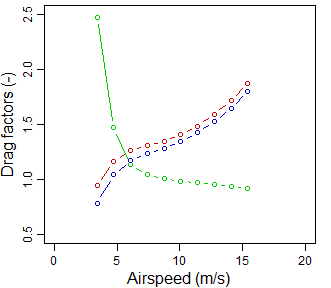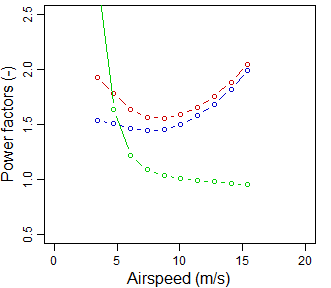The above figure shows the speed dependency of the drag factors and power factors. At low speeds the lift-dependent drag factors have a low value. There is even a tendency for the factors to drop below 1. The zero-lift profile drag instead takes very large values. At these low speeds the velocity of the wings starts to become more dominant than the flight speed.

### miscellaneous

Several other variables are also stored in table:

flightperf$table[c('frequency','L','ReynoldsNumber','CDpro0')] ## frequency L ReynoldsNumber CDpro0 ## minimumSpeed 5 2.10843 22646.05 0.017676057 ## minimumPower 5 2.10843 54284.31 0.011416802 ## maximumRange 5 2.10843 74048.32 0.009775163 ## maximumSpeed 5 2.10843 102868.70 0.008293541 These are respectively the wingbeat frequency (in this case as specified in myBird), the lift force (in this case just equal to weight), the mean chord Reynolds number, and the zero lift profile drag coefficient (dependent on mean chord Reynolds number). ## Flight condition All models by default use the internal FLIGHTCONDITION, which describes the standard atmosphere, with air density $$\rho=1.225$$ kg m3, gravitational acceleration $$g=9.81$$ m s-2 and kinematic viscosity $$\nu=14.61\times 10^{-6}$$ m2 s-1. It is possible to define an alternative flight condition: myFlightCondition <- list( density = 0.9093, # [kg/m3] 3 km altitude gravity = 9.81, # [m/s2] viscosity = 18.63E-6, # [m2/s] 3 km altitude windSpeed = 0,# [m/s] windDir = 0 # [degrees] 0 degrees means wind is along the track direction ) Air density decreases with altitude, and a density of $$\rho=0.909$$ kg m3 corresponds to a flight altitude of 3 km above sea level in the international standard atmosphere. At this altitude the kinematic viscosity has increased to $$\nu=18.63\times 10^{-6}$$ m2 s-1. This alternative flight condition can be used in the performance calculation as: flightperf.ISA3 <- computeFlightPerformance(myBird,flightcondition=myFlightCondition) flightperf.ISA3 ## Name: Jackdaw ## Sc. name: Corvus monedula ## Bird definitions: KleinHeerenbrink M, Warfvinge K and Hedenstrom A (2016) J.Exp.Biol. 219: 10, 1572--1581 ## speed power.aero power.chem strokeplane amplitude ## minimumSpeed 4.946 4.531 23.93 46.2 54.4 ## minimumPower 9.556 2.634 14.85 22.8 40.7 ## maximumRange 12.873 3.129 17.22 15.6 43.5 ## maximumSpeed 16.372 4.531 23.93 11.5 51.1 ## Maximum climb performance: ## speed power.aero power.chem strokeplane amplitude climbRate ## maximumClimbRate 8.886 4.531 23.93 22.6 53.5 0.8587 ## Minimized migration time: ## speed speed.migration power.aero power.chem power.dep ## minimumTimeSpeed 13.57 1.961 3.339 18.23 3.081 ## strokeplane amplitude ## minimumTimeSpeed 14.56 44.79 Note how the power requirements have increased (this is only apparent from the minimumPower and maximumRange, as the maximum available power is unchanged), but the characteristic speeds also increased. Looking at the energetic cost per traveled distance: COT.ISA0 <- with(flightperf$table,power.chem/speed)
COT.ISA3 <-with(flightperf.ISA3$table,power.chem/speed) list(ISA0 = COT.ISA0, ISA3 = COT.ISA3) ##$ISA0
##  7.044379 1.549928 1.323949 1.550427
##
## \$ISA3
##  4.838110 1.554379 1.337836 1.461550
COT.ISA3/COT.ISA0
##  0.6868043 1.0028719 1.0104890 0.9426758

it appears that flying at the maximum range speed at a higher altitude will actually reduce the maximum range (which can be attributed to the increase in kinematic viscosity, increasing the profile drag coefficient). However, the 1% increase in flight cost does pay back as a 15% reduction in flight time. In fact, if the bird cares about time minimization, flying at altitude is highly advantageous, increasing the maximum flight speed while reducing the associated cost of transport.

1. At the minimum speed, the assumption of fast forward flight may be invalid. See also the section about validity flags.

2. see Klein Heerenbrink et al. (2015) p. 13.

3. flapping flight factors as per Klein Heerenbrink et al. (2015).